Online Tution   »   CBSE Syllabus - Class 9,10, 11,...   »   CBSE Class 12 Physics Syllabus 2022-23...

## Class 12 Physics Syllabus 2022-23

CBSE Class 12 physics syllabus 2022-23 has been released on the official website and students can download the syllabus in PDF format. In this article, we have included all the units with mark distribution and several periods to complete the portion.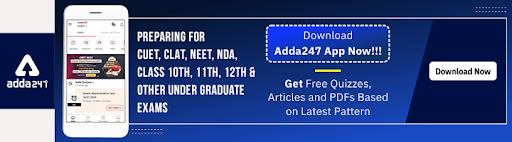We have also included the question paper design and the class 12 physics syllabus with marks distribution. Class 12th is the final stage of school life. After 12th students have to go for higher studies.

Class 12 Physics Important Question Chapter wise 2023

Class 12 Physics Previous Year Papers 2023

Class 12 Physics Important MCQs 2023

The class 12 physics syllabus will help the students to keep a check on the completed portion and the incompleted portion so that they can manage their time accordingly and set their study timetable.

## Physics Syllabus CBSE Class 12 2022-23

CBSE class 12 physics syllabus 2022-23, is available on the official website of CBSE for the students. CBSE class 12 physics syllabus 2022-23 has 9 units, electrostatics, current electricity, magnetic effect of current and magnetism, electromagnetic induction and alternating current, electromagnetic waves, optics, dual nature of radiation and matter, atoms and nuclei, electronic devices.

If the students prepare well and start with a detailed view of CBSE class 12th physics syllabus 2022-23, They can score good marks.Below the given table is an expanded view of CBSE class 12th physics 2022-23 units with marks distribution and several periods.

 Units Name of the unit No. of Periods Marks Unit–I Electrostatics 26 16 Unit-II Current Electricity 18 Unit-III Magnetic Effects of Current and Magnetism 25 17 Unit-IV Electromagnetic Induction and Alternating Currents 24 Unit–V Electromagnetic Waves 04 18 Unit–VI Optics 30 Unit–VII Dual Nature of Radiation and Matter 8 12 Unit–VIII Atoms and Nuclei 15 Unit–IX Electronic Devices 10 7 Total 160 70 Practicals 30 Total 100

## Class 12 Physics Syllabus 2022-23 & Marking Scheme

Physics is a very vast subject and students can take physics as their as the main subject for higher studies. Students who are preparing for the competitive exams must go through the syllabus for classes 12th and 11th.

The theory paper is 70 marks and the practical exam is 30 marks together with the CBSE class 12 exam is 100 marks. Of all the units Unit 5 which is electromagnetic waves and unit 6 which is optics carry the highest marks which are 18 out of 70. Students do not have to worry about 9 units because all the units have two maximum chapters.

CBSE Class 12 Physics syllabus 2022-23 PDF is given below.

## CBSE Class 12 Physics Syllabus 2022-23- Unit-wise Summary

CBSE Class 12 Physics Syllabus 2022-23 unit-wise detailed syllabus is given below.

## Class 12 Physics Syllabus 2022-23 Unit 1- Unit I: Electrostatics

CBSE Class 12 Physics syllabus 2022-23: Unit wise syllabus is given below. check full details.

• Chapter–1: Electric Charges and Fields

Electric charges, Conservation of charge, Coulomb’s law-force between two point charges, forces between multiple charges; superposition principle, and continuous charge distribution.

Electric field, electric field due to a point charge, electric field lines, electric dipole, electric field due to a dipole, torque on a dipole in a uniform electric field.

Electric flux is, a statement of Gauss’s theorem and its applications to find fields due to infinitely long straight wire, uniformly charged infinite plane sheet, and uniformly charged thin spherical shell (field inside and outside).

• Chapter–2: Electrostatic Potential and Capacitance

Electric potential, potential difference, electric potential due to a point charge, a dipole and system of charges; equipotential surfaces, the electrical potential energy of a system of two point charges, and electric dipole in an electrostatic field.

Conductors and insulators, free charges, and bound charges inside a conductor. Dielectrics and electric polarization, capacitors and capacitance, a combination of capacitors in series and parallel, capacitance of a parallel plate capacitor with and without dielectric medium between the plates, energy stored in a capacitor (no derivation, formulae only).

## Class 12 Physics Syllabus 2022-23 Unit 2: Current Electricity

Chapter–3: Current Electricity

Electric current, the flow of electric charges in a metallic conductor, drift velocity, mobility and their relation with electric current; Ohm’s law, V-I characteristics (linear and non-linear), electrical energy and power, electrical resistivity and conductivity, the temperature dependence of resistance, Internal resistance of a cell, potential difference and emf of a cell, a combination of cells in series and parallel, Kirchhoff’s rules, Wheatstone bridge.

## Class 12 Physics Syllabus 2022-23 Unit 3: Magnetic Effects of Current and Magnetism

• Chapter–4: Moving Charges and Magnetism

Concept of the magnetic field, Oersted’s experiment. Biot – Savart law and its application to current carrying circular loop. Ampere’s law and its applications to an infinitely long straight wire. Straight solenoid (only qualitative treatment), force on a moving charge in uniform magnetic and electric fields.

Force on a current-carrying conductor in a uniform magnetic field, the force between two parallel current-carrying conductors-definition of an ampere, torque experienced by a current loop in a uniform magnetic field; Current loop as a magnetic dipole and its magnetic dipole moment, moving coil galvanometers current sensitivity and conversion to ammeter and voltmeter.

• Chapter–5: Magnetism and Matter

Bar magnet, bar magnet as an equivalent solenoid (qualitative treatment only), magnetic field intensity due to a magnetic dipole (bar magnet) along its axis and perpendicular to its axis (qualitative treatment only), torque on a magnetic dipole (bar magnet) in a uniform magnetic field (qualitative treatment only), magnetic field lines.

Magnetic properties of materials- Para-, dia- and ferromagnetic substances with examples, Magnetization of materials, the effect of temperature on magnetic properties.

## Class 12 Physics Syllabus 2022-23 Unit 4: Electromagnetic Induction and Alternating Currents

• Chapter–6: Electromagnetic Induction

Electromagnetic induction; Faraday’s laws, induced EMF and current; Lenz’s Law, Self, and mutual induction.

• Chapter–7: Alternating Current

Alternating currents, peak and RMS value of alternating current/voltage; reactance and impedance; LCR series circuit (phasors only), resonance, power in AC circuits, power factor, wattless current.

AC generator, Transformer.

## Class 12 Physics Syllabus 2022-23 Unit 5: Electromagnetic waves

• Chapter–8: Electromagnetic Waves

The basic idea of displacement current, Electromagnetic waves, their characteristics, their transverse nature (qualitative idea only).

Electromagnetic spectrum (radio waves, microwaves, infrared, visible, ultraviolet, X-rays, gamma rays) includes elementary facts about their uses.

## Class 12 Physics Syllabus 2022-23 Unit VI: Optics

• Chapter–9: Ray Optics and Optical Instruments

Ray Optics: Reflection of light, spherical mirrors, mirror formula, refraction of light, total internal reflection and optical fibers, refraction at spherical surfaces, lenses, thin lens formula, lens maker’s formula, magnification, power of a lens, a combination of thin lenses in contact, refraction of light through a prism.

Optical instruments: Microscopes and astronomical telescopes (reflecting and refracting) and their magnifying powers.

• Chapter–10: Wave Optics

Wave optics: Wavefront and Huygen’s principle, reflection, and refraction of plane wave at a plane surface using wavefronts. Proof of laws of reflection and refraction using Huygen’s principle. Interference, Young’s double-slit experiment and expression for fringe width (No derivation final expression only), coherent sources and sustained interference of light, diffraction due to a single slit, width of central maxima (qualitative treatment only).

## Class 12 Physics Syllabus 2022-23 Unit VII:   Dual Nature of Radiation and Matter 08 Periods

• Chapter–11: Dual Nature of Radiation and Matter

Dual nature of radiation, Photoelectric effect, Hertz and Lenard’s

observations; Einstein’s photoelectric equation-particle nature of light.

Experimental study of the photoelectric effect

Matter waves-wave nature of particles, de-Broglie relation.

## Class 12 Physics Syllabus 2022-23 Unit 8:   Atoms and Nuclei

• Chapter–12: Atoms

Alpha-particle scattering experiment; Rutherford’s model of atom; Bohr model of the hydrogen atom, Expression for the radius of the nth possible orbit, velocity and energy of an electron in his orbit, of hydrogen line spectra (qualitative treatment only).

• Chapter–13: Nuclei

Composition and size of nucleus, nuclear force

Mass-energy relation, mass defect; binding energy per nucleon and its variation with mass number; nuclear fission, nuclear fusion.

## Class 12 Physics Syllabus 2022-23 Unit 9: Electronic Devices

• Chapter–14: Semiconductor Electronics: Materials, Devices, and Simple Circuits

Energy bands in conductors, semiconductors, and insulators (qualitative ideas only) Intrinsic and extrinsic semiconductors- p and n-type, p-n junction

Semiconductor diode – I-V characteristics in forward and reverse bias, application of junction diode -diode as a rectifier.

## Class 12 Physics Syllabus 2022-23: Practicals

CBSE class 12 physics practical exams are 30 marks. These 30 marks are divided into 5 parts, two experiments, a practical record, an activity, an investigatory project, and viva voce. There are two sections of 10 experiments from which students have two complete two experiments during the exam. Store students have to submit their practical records before the exam. Below given table and the further details will tell the students about the practical exams thoroughly.

### CBSE Class 12 Physics Syllabus 2022-23: Practicals

 CBSE Class 12 Physics Syllabus 2022-23: Practicals Two experiments one from each section 7+7 Marks Practical record [experiments and activities] 5 Marks One activity from any section 3 Marks Investigatory Project 3 Marks Viva on experiments, activities, and project 5 Marks Total 30 marks

List of Practicals and Activities performed by the students

SECTION–A: Experiments

1. To determine the resistivity of two / three wires by plotting a graph for potential difference versus current.
2. To find the resistance of a given wire / standard resistor using a meter bridge.
3. To verify the laws of combination (series) of resistances using a meter bridge.

OR

To verify the laws of combination (parallel) of resistances using a meter bridge.

1. To determine the resistance of a galvanometer by half-deflection method and to find its figure of merit.
2. To convert the given galvanometer (of known resistance and figure of merit) into a voltmeter of desired range and to verify the same.

OR

To convert the given galvanometer (of known resistance and figure of merit) into an ammeter of desired range and to verify the same.

1. To find the frequency of AC mains with a sonometer.

Activities

1. To measure the resistance and impedance of an inductor with or without an iron core.
2. To measure resistance, voltage (AC/DC), current (AC), and check continuity of a given circuit using a multimeter.
3. To assemble a household circuit comprising three bulbs, three (on/off) switches, a

Fuse, and a power source.

1. To assemble the components of a given electrical circuit.
2. To study the variation in potential drop with the length of a wire for a steady current.
3. To draw the diagram of a given open circuit comprising at least a battery, resistor/rheostat, key, ammeter, and voltmeter. Mark the components that are not connected in proper order and correct the circuit and also the circuit diagram.

SECTION-B: Experiments

1. To find the value of v for different values of u in the case of a concave mirror and to find the focal length.
2. To find the focal length of a convex mirror, using a convex lens.
3. To find the focal length of a convex lens by plotting graphs between u and v or between 1/u and 1/v.
4. To find the focal length of a concave lens, using a convex lens.
5. To determine the angle of minimum deviation for a given prism by plotting a graph between the angle of incidence and the angle of deviation.
6. To determine the refractive index of a glass slab using a traveling microscope.
7. To find the refractive index of a liquid using the convex lens and plane mirror.
8. To find the refractive index of a liquid using a concave mirror and a plane mirror.
9. To draw the I-V characteristic curve for a p-n junction diode in forward and reverse bias.

Activities

1. To identify a diode, an LED, a resistor, and a capacitor from a mixed collection of such items.
2. Use of multimeter to see the unidirectional flow of current in the case of a diode and an LED and check whether a given electronic component (e.g., diode) is in working order.
3. To study the effect of intensity of light (by varying distance of the source) on an LDR.
4. To observe refraction and lateral deviation of a beam of light incident obliquely on a glass slab.
5. To observe the diffraction of light due to a thin slit.
6. To study the nature and size of the image formed by an (i) convex lens, or (ii) concave mirror, on a screen by using a candle and a screen (for different distances of the candle from the lens/mirror).
7. To obtain a lens combination with the specified focal length by using two lenses from the given set of lenses.

## Class 12 Physics Syllabus 2022-23: Suggested Investigatory Projects

1. To study various factors on which the internal resistance/EMF of a cell depends.
2. To study the variations in current flowing in a circuit containing an LDR because of a variation in

(a) the power of the incandescent lamp used to ‘illuminate’ the LDR (keeping all the lamps at a fixed distance).

(b) the distance of an incandescent lamp (of fixed power) used to ‘illuminate’ the LDR.

1. To find the refractive indices of (a) water (b) oil (transparent) using a plane mirror, a biconvex lens (made from a glass of known refractive index), and an adjustable object needle.
2. To investigate the relation between the ratio of (i) output and input voltage and (ii) number of turns in the secondary coil and primary coil of a self-designed transformer.
3. To investigate the dependence of the angle of deviation on the angle of incidence using a hollow prism filled one by one, with different transparent fluids.
4. To estimate the charge induced on each one of the two identical Styrofoam (or pith) balls suspended in a vertical plane by making use of Coulomb’s law.
5. To study the factor on which the self-inductance of a coil depends by observing the effect of this coil when put in series with a resistor/(bulb) in a circuit fed up by an A.C. source of adjustable frequency.
6. To study the earth’s magnetic field using a compass needle-bar magnet by plotting magnetic field lines and tangent galvanometer.

## Class 12 Physics Syllabus 2022-23: Practicals For Visually Impaired

1. Items for Identification/ familiarity with the apparatus for assessment in practicals (All experiments)

Meter scale, the general shape of the voltmeter/ammeter, battery/power supply, connecting wires, standard resistances, connecting wires, voltmeter/ammeter, meter bridge, screw gauge, jockey Galvanometer, Resistance Box, standard Resistance, connecting wires, Potentiometer, jockey, Galvanometer, Lechlanche cell, Daniell cell [simple distinction between the two vis-à-vis their outer (glass and copper) containers], rheostat connecting wires, Galvanometer, resistance box, Plug-in and tapping keys, connecting wires battery/power supply,  Diode,  Resistor (Wire-wound or carbon ones with two wires connected to two ends), capacitors (one or two types), Inductors, Simple electric/electronic bell, battery/power supply, Plugin and tapping keys, Convex lens, concave lens, convex mirror, concave mirror, Core/hollow wooden cylinder, insulated wire, ferromagnetic rod, Transformer core, insulated wire.

List of Practicals

1. To determine the resistance per cm of a given wire by plotting a graph between voltage and current.
2. To verify the laws of combination (series/parallel combination) of resistances by

Ohm’s law.

1. To find the resistance of a given wire / standard resistor using a meter bridge.
2. To determine the resistance of a galvanometer by half deflection method.
3. To identify a resistor, capacitor, inductor, and diode from a mixed collection of such items.
4. To observe the difference between

(i) a convex lens and a concave lens

(ii) a convex mirror and a concave mirror and to estimate the likely difference between the power of two given convex /concave lenses.

1. To design an inductor coil and to know the effect of

(i) change in the number of turns

(ii) Introduction of the ferromagnetic material as its core material on the inductance of the coil.

1. To design an (i) step up (ii) step down transformer on a given core and know the relation between its input and output voltages.

## Class 12 Physics 2022-23:  Question Paper Design According Syllabus

 S Typology of Questions Total Marks Approximate Percentage 1 Remembering Understanding 27 38 % 2 Applying 22 32% 3 Analyzing Evaluating Creating 21 30% Total Marks 70 100 Practical 30 100 Gross Total

## Class 12 Physics Syllabus 2022-23: Reference Books

Sharing is caring!

## FAQs

### How can I get CBSE Class 12 physics Syllabus Pdf?

The CBSE Class 12 physics Syllabus pdf is given on this article. You can download the CBSE Class 12 physics Syllabus in Pdf format,

### What are the reference books for CBSE Class 12 physics?

The reference books for CBSE Class 12 physics are:

•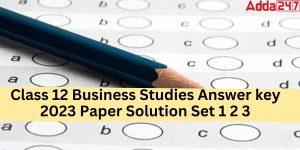Class 12 Business Studies Answer key 202...
•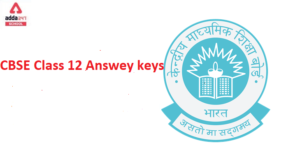CBSE Class 12 Answer Key (All Subjects) ...
•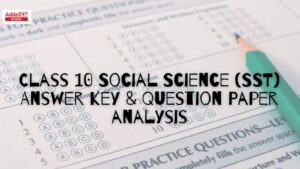CBSE Class 10 SST Answer key 2023, Socia...
•CBSE Class 12 Informatics Practices Syll...
•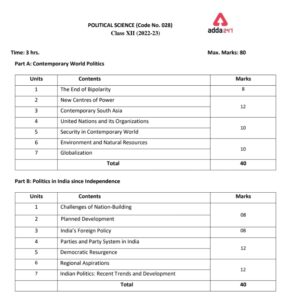Political Science Class 12 Syllabus 2022...
•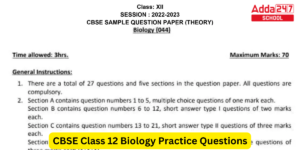CBSE Class 12 Biology Additional Practic...